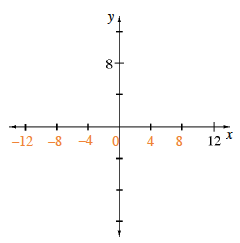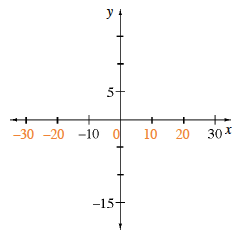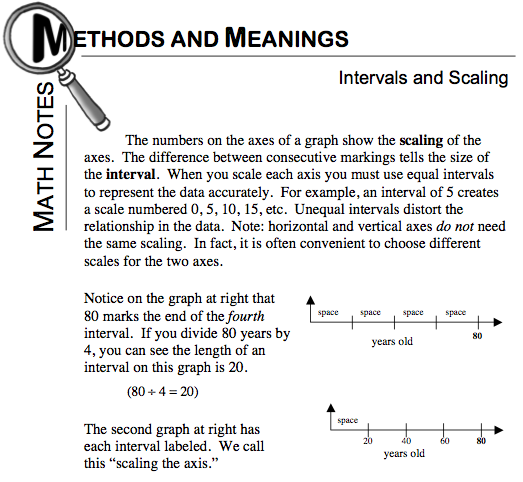### Home > CCAA > Chapter 9 Unit 8 > Lesson CC2: 9.1.1 > Problem9-13

9-13.

Copy the axes below and complete the scales.  Homework Help ✎

For a review of scaling, refer to the Math Notes box below.

Remember that where the $x\text{-}$ and $y\text{-axes}$ intersect is always $0$. To scale the $x\text{-axis}$ in part (a), you should try dividing $12$ by $3$, since that is the number of spaces between $0$ and $12$.

1.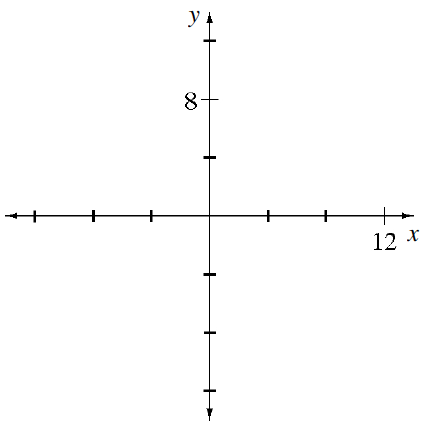1.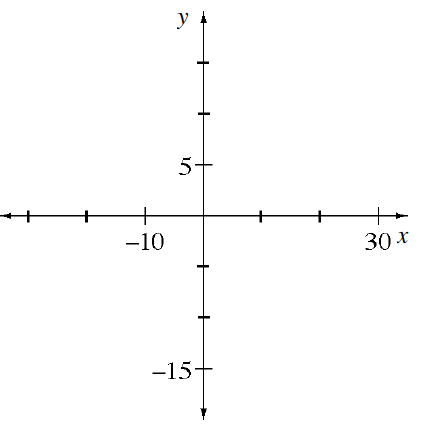The $x\text{-axes}$ for parts (a) and (b) are labeled below. Were you able to scale the $y\text{-axes}$?